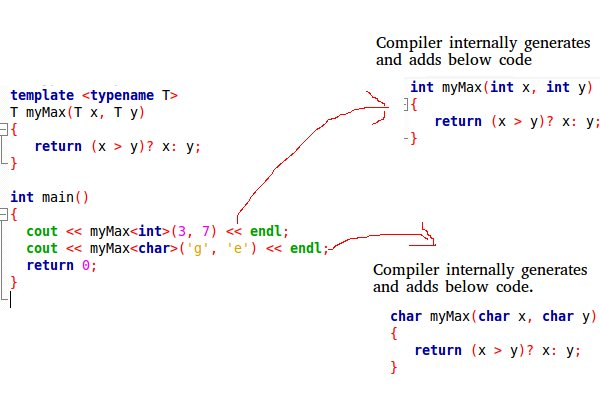# Templates in C++

• Difficulty Level : Medium
• Last Updated : 28 Jun, 2021

A template is a simple and yet very powerful tool in C++. The simple idea is to pass data type as a parameter so that we don’t need to write the same code for different data types. For example, a software company may need sort() for different data types. Rather than writing and maintaining the multiple codes, we can write one sort() and pass data type as a parameter.
C++ adds two new keywords to support templates: ‘template’ and ‘typename’. The second keyword can always be replaced by keyword ‘class’.
How do templates work?
Templates are expanded at compiler time. This is like macros. The difference is, the compiler does type checking before template expansion. The idea is simple, source code contains only function/class, but compiled code may contain multiple copies of same function/class.Want to learn from the best curated videos and practice problems, check out the C++ Foundation Course for Basic to Advanced C++ and C++ STL Course for foundation plus STL.  To complete your preparation from learning a language to DS Algo and many more,  please refer Complete Interview Preparation Course.

Function Templates We write a generic function that can be used for different data types. Examples of function templates are sort(), max(), min(), printArray().
Know more on Generics in C++

## CPP

 `#include ``using` `namespace` `std;` `// One function works for all data types.  This would work``// even for user defined types if operator '>' is overloaded``template` `<``typename` `T>``T myMax(T x, T y)``{``   ``return` `(x > y)? x: y;``}` `int` `main()``{``  ``cout << myMax<``int``>(3, 7) << endl;  ``// Call myMax for int``  ``cout << myMax<``double``>(3.0, 7.0) << endl; ``// call myMax for double``  ``cout << myMax<``char``>(``'g'``, ``'e'``) << endl;   ``// call myMax for char` `  ``return` `0;``}`

Output:

```7
7
g```

Below is the program to implement Bubble Sort using templates in C++:

## CPP

 `// CPP code for bubble sort``// using template function``#include ``using` `namespace` `std;`` ` `// A template function to implement bubble sort.``// We can use this for any data type that supports``// comparison operator < and swap works for it.``template` `<``class` `T>``void` `bubbleSort(T a[], ``int` `n) {``    ``for` `(``int` `i = 0; i < n - 1; i++)``        ``for` `(``int` `j = n - 1; i < j; j--)``            ``if` `(a[j] < a[j - 1])``              ``swap(a[j], a[j - 1]);``}`` ` `// Driver Code``int` `main() {``    ``int` `a = {10, 50, 30, 40, 20};``    ``int` `n = ``sizeof``(a) / ``sizeof``(a);`` ` `    ``// calls template function``    ``bubbleSort<``int``>(a, n);`` ` `    ``cout << ``" Sorted array : "``;``    ``for` `(``int` `i = 0; i < n; i++)``        ``cout << a[i] << ``" "``;``    ``cout << endl;`` ` `  ``return` `0;``}`

Output

`Sorted array : 10 20 30 40 50`

Class Templates Like function templates, class templates are useful when a class defines something that is independent of the data type. Can be useful for classes like LinkedList, BinaryTree, Stack, Queue, Array, etc.
Following is a simple example of template Array class.

## CPP

 `#include ``using` `namespace` `std;` `template` `<``typename` `T>``class` `Array {``private``:``    ``T *ptr;``    ``int` `size;``public``:``    ``Array(T arr[], ``int` `s);``    ``void` `print();``};` `template` `<``typename` `T>``Array::Array(T arr[], ``int` `s) {``    ``ptr = ``new` `T[s];``    ``size = s;``    ``for``(``int` `i = 0; i < size; i++)``        ``ptr[i] = arr[i];``}` `template` `<``typename` `T>``void` `Array::print() {``    ``for` `(``int` `i = 0; i < size; i++)``        ``cout<<``" "``<<*(ptr + i);``    ``cout< a(arr, 5);``    ``a.print();``    ``return` `0;``}`

Output:

` 1 2 3 4 5`

Can there be more than one arguments to templates?
Yes, like normal parameters, we can pass more than one data types as arguments to templates. The following example demonstrates the same.

## CPP

 `#include``using` `namespace` `std;` `template``<``class` `T, ``class` `U>``class` `A  {``    ``T x;``    ``U y;``public``:``    ``A() {    cout<<``"Constructor Called"``< a;``   ``A<``int``, ``double``> b;``   ``return` `0;``}`

Output:

```Constructor Called
Constructor Called```

Can we specify default value for template arguments?
Yes, like normal parameters, we can specify default arguments to templates. The following example demonstrates the same.

## CPP

 `#include``using` `namespace` `std;` `template``<``class` `T, ``class` `U = ``char``>``class` `A  {``public``:``    ``T x;``    ``U y;``    ``A() {   cout<<``"Constructor Called"``< a;  ``// This will call A  ``   ``return` `0;``}`

Output:

`Constructor Called`

Both function overloading and templates are examples of polymorphism feature of OOP. Function overloading is used when multiple functions do similar operations, templates are used when multiple functions do identical operations.
What happens when there is a static member in a template class/function?
Each instance of a template contains its own static variable. See Templates and Static variables for more details.
What is template specialization?
Template specialization allows us to have different code for a particular data type. See Template Specialization for more details.
Can we pass nontype parameters to templates?
We can pass non-type arguments to templates. Non-type parameters are mainly used for specifying max or min values or any other constant value for a particular instance of a template. The important thing to note about non-type parameters is, they must be const. The compiler must know the value of non-type parameters at compile time. Because the compiler needs to create functions/classes for a specified non-type value at compile time. In below program, if we replace 10000 or 25 with a variable, we get a compiler error. Please see this.
Below is a C++ program.

## CPP

 `// A C++ program to demonstrate working of non-type``// parameters to templates in C++.``#include ``using` `namespace` `std;`` ` `template` `<``class` `T, ``int` `max>``int` `arrMin(T arr[], ``int` `n)``{``   ``int` `m = max;``   ``for` `(``int` `i = 0; i < n; i++)``      ``if` `(arr[i] < m)``         ``m = arr[i];`` ` `   ``return` `m;``}`` ` `int` `main()``{``   ``int` `arr1[]  = {10, 20, 15, 12};``   ``int` `n1 = ``sizeof``(arr1)/``sizeof``(arr1);`` ` `   ``char` `arr2[] = {1, 2, 3};``   ``int` `n2 = ``sizeof``(arr2)/``sizeof``(arr2);`` ` `   ``// Second template parameter to arrMin must be a constant``   ``cout << arrMin<``int``, 10000>(arr1, n1) << endl;``   ``cout << arrMin<``char``, 256>(arr2, n2);``   ``return` `0;``}`

Output:

```10
1```

What is template metaprogramming?
See Template Metaprogramming
You may also like to take a quiz on templates.
Java also supports these features. Java calls it generics .